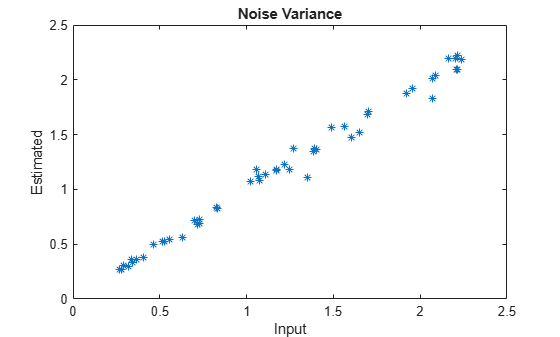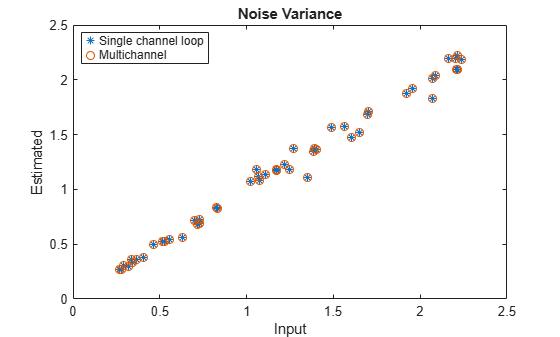# armcov

Autoregressive all-pole model parameters — modified covariance method

## Syntax

``a = armcov(x,p)``
``[a,e] = armcov(x,p)``

## Description

example

````a = armcov(x,p)` returns the normalized autoregressive (AR) parameters corresponding to a model of order `p` for the input array `x`. `x` is assumed to be the output of an AR system driven by white noise. This method minimizes the forward and backward prediction errors in the least-squares sense```
````[a,e] = armcov(x,p)` also returns the estimated variance, `e`, of the white noise input.```

## Examples

collapse all

Use a vector of polynomial coefficients to generate an AR(4) process by filtering 1024 samples of white noise. Reset the random number generator for reproducible results. Use the modified covariance method to estimate the coefficients.

```rng default A = [1 -2.7607 3.8106 -2.6535 0.9238]; y = filter(1,A,0.2*randn(1024,1)); arcoeffs = armcov(y,4)```
```arcoeffs = 1×5 1.0000 -2.7741 3.8404 -2.6841 0.9360 ```

Generate 50 realizations of the process, changing each time the variance of the input noise. Compare the modified-covariance-estimated variances to the actual values.

```nrealiz = 50; noisestdz = rand(1,nrealiz)+0.5; randnoise = randn(1024,nrealiz); noisevar = zeros(1,nrealiz); for k = 1:nrealiz y = filter(1,A,noisestdz(k) * randnoise(:,k)); [arcoeffs,noisevar(k)] = armcov(y,4); end plot(noisestdz.^2,noisevar,'*') title('Noise Variance') xlabel('Input') ylabel('Estimated')```Repeat the procedure using the function's multichannel syntax.

```Y = filter(1,A,noisestdz.*randnoise); [coeffs,variances] = armcov(Y,4); hold on plot(noisestdz.^2,variances,'o') hold off legend('Single channel loop','Multichannel','Location',"best")```## Input Arguments

collapse all

Input array, specified as a vector or matrix.

Example: `filter(1,[1 -0.75 0.5],0.2*randn(1024,1))` specifies a second-order autoregressive process.

Data Types: `single` | `double`
Complex Number Support: Yes

Model order, specified as a positive integer scalar. `p` must be less than the number of elements or rows of `x`.

Data Types: `single` | `double`

## Output Arguments

collapse all

Normalized autoregressive parameters, returned as a vector or matrix. If `x` is a matrix, then each row of `a` corresponds to a column of `x`. `a` has `p` + 1 columns and contains the AR system parameters, A(z), in descending powers of z.

White noise input variance, returned as a scalar or row vector. If `x` is a matrix, then each element of `e` corresponds to a column of `x`.

collapse all

### AR(p) Model

In an AR model of order p, the current output is a linear combination of the past p outputs plus a white noise input. The weights on the p past outputs minimize the mean squared prediction error of the autoregression.

Let y(n) be a wide-sense stationary random process obtained by filtering white noise of variance e with the system function A(z). If Py(e) is the power spectral density of y(n), then

`${P}_{y}\left({e}^{j\omega }\right)=\frac{e}{{|A\left({e}^{j\omega }\right)|}^{2}}=\frac{e}{{|1+\sum _{k=1}^{p}a\left(k\right){e}^{-j\omega k}|}^{2}}.$`

Because the modified covariance method characterizes the input data using an all-pole model, the correct choice of the model order, p, is important.

## Version History

Introduced before R2006a